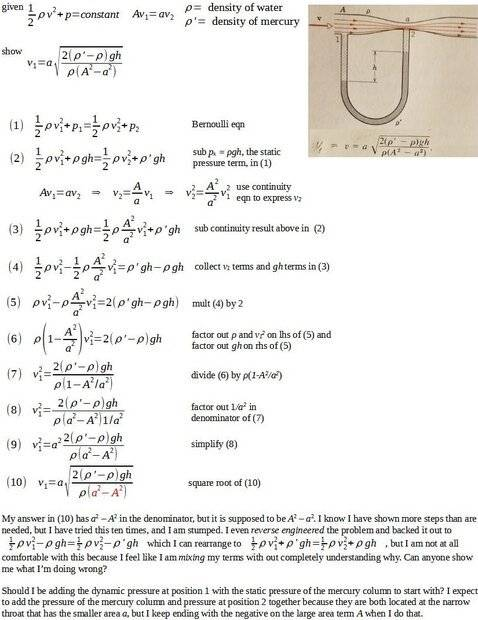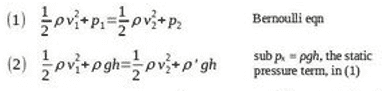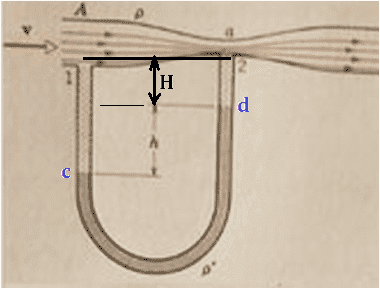# Derive the Venturi Meter eqn from the Bernoulli eqn

Gold Member
Homework Statement:
By applying Bernoulli's equation and the equation of continuity to points 1 and 2 of Fig. 16-14 [see attached file], show that the speed of the flow at the entrance is
v1 = a*sqrt{(2(dens' - dens)gh)/(dens(A^2-a^2))}
Relevant Equations:
0.5*dens*v_1^2 + p_1 = 0.5*dens*v_2^2 + p_2 Bernoulii eqn
A*v_1 = a*v_2 continuity eqn
Advanced apologies for this format; I am posting my question as an the image b/c the Latex is being very buggy with me, and I lost a kind of lengthy post to it. Can anyone show me what I am doing wrong? I have attached a pdf version for easier reading if need be.#### Attachments

• pr-43-p-290-h-r-text-ed.pdf
102.1 KB · Views: 12

Homework Helper
Gold MemberFrom equation (1) you can see that ##p_2## must be less than ##p_1## because ##v_2 > v_1## (from the continuity equation). So, ##p_2 < p_1##.

However, in equation (2) you let ##p_1 = \rho g h## and ##p_2 =\rho' \, gh##.
But ##\rho' \, > \rho##. So, these substitutions would imply that ##p_2 > p_1##, which contradicts ##p_2 < p_1##. So, letting ##p_1 = \rho g h## and ##p_2 =\rho' \, gh## can't be correct.

Assume we can take points 1 and 2 to be at the same horizontal level:Introduce the height ##H## as shown. Can you express ##p_1## in terms of ##p_c## , ##\rho##, ##g##, ##H##, and ##h##? Likewise, can you relate ##p_2## and ##p_d##?

Gold Member
Thnx so much for the response TNsy. Pointing out my contradiction between lines (1) and (2) was the big aha moment for me, and including the ##\rho gH## term makes the physical sense clear now.

•TSny